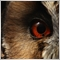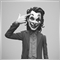# StopLoss and TakeProfit with Bid and Ask64

Hi All,

sorry for basic question,

CTrade::Buy(const double volume,const string symbol=NULL,double price=0.0,const double sl=0.0,const double tp=0.0,const string comment="")

The below parameters for Sell

bool CTrade::Sell(const double volume,const string symbol=NULL,double price=0.0,const double sl=0.0,const double tp=0.0,const string comment="")

Is the below usage of Bid and Ask correct ?

let x and y be some double value

takeprofit Bid+y

price Bid

stoploss Bid+x

takeprofit Bid-y15291

quantumninja:

Is the below usage of Bid and Ask correct ?

let x and y be some double value

takeprofit Bid+y

price Bid

stoploss Bid+x

takeprofit Bid-y

technically correct, as long as the price (stoploss or Takeprofit value) not within StopLevel limit.
but, for better calculation of pip distance in point;

Stoploss = Bid-x
TakeProfit = Bid+y

Sell:

but, it all depends on you as a coder.19998

quantumninja: Is the below usage of Bid and Ask correct ?

takeprofit Bid+y

price Bid

stoploss Bid+x

takeprofit Bid-y